# C program to find the largest among three numbers

This article will teach you how to find the largest or greatest number among three numbers given by the user (at run-time) using and without the ternary operator. Here are the approaches you will go through:

## Using if-else-if logic, find the largest of the three numbers

To find the largest number among three given numbers in C programming, first ask the user to enter any three numbers, then use the if-else block to determine which one is the largest, as shown in the program below:

```#include<stdio.h>
#include<conio.h>
int main()
{
int a, b, c, big;
printf("Enter three numbers: ");
scanf("%d%d%d", &a, &b, &c);
if(a>b)
{
if(b>c)
big = a;
else
{
if(c>a)
big = c;
else
big = a;
}
}
else
{
if(b>c)
big = b;
else
big = c;
}
printf("\nLargest number = %d", big);
getch();
return 0;
}```

Because the above program was written in the Code::Blocks IDE, you will receive the following output after a successful build and run: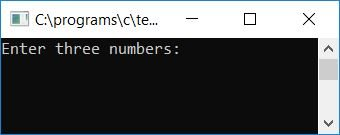Supply any three numbers, say 3, 1, and 2. Press the ENTER key to see the largest number as given in the second snapshot of the sample run: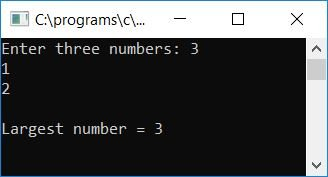Here is another sample run: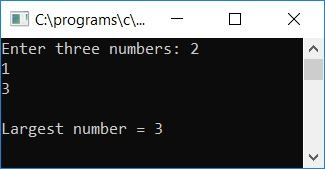And here is the last sample run of the above program: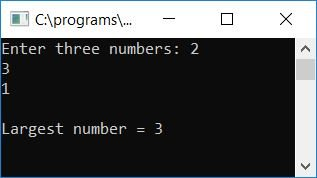Here are the cases in which the user can enter the three numbers:

• The first number is the largest, and the second number is the second largest.
• The first number is the biggest, and the third number is the second biggest.
• The second number is the largest, and the first number is the second largest.
• The second number is the largest, and the third number is the second largest.
• The third number is the biggest, and the first number is the second biggest.
• The third number is the largest, and the second number is the second largest.

#### Program Explained

• Receive three numbers as input and store them inside the three variables as the first, second, and third numbers, say a, b, and c.
• Check whether the first number, say a, is greater than the second number, say b.
• If it is, then go to the if block and check whether the value inside b is also greater than c.
• If it is, then the value present at the first number, say a, will be initialized to the variable big; otherwise, check whether the third number, say c, is greater than the first number, say a.
• If it is, then the value present at c gets initialized to big; otherwise, initialize a to big and come out of the if block.
• And if a is less than b, then check whether b is greater than c.
• If it is, initialize the value of b to big; otherwise, initialize the value of c to big.
• Print the value of the big variable as output; that will be the largest value among the given three numbers.

## Using if-else logic, find the largest of three numbers.Statement

This program also does the same job but in a simple manner, using an if-else statement in the C language:

```#include<stdio.h>
#include<conio.h>
int main()
{
int a, b, c, big;
printf("Enter three numbers: ");
scanf("%d%d%d", &a, &b, &c);
if(a>b && a>c)
big = a;
else if(b>a && b>c)
big = b;
else
big = c;
printf("\nLargest number = %d", big);
getch();
return 0;
}```

The above program is a little simpler to create and understand in comparison to the above one. You will see the same output as the previous one.

## Using the ternary operator, find the largest of three numbers

Again, I'm going to create the same program using the ternary operator (?:).

```#include<stdio.h>
#include<conio.h>
int main()
{
int num1, num2, num3, large;
printf("Enter any three numbers: ");
scanf("%d%d%d", &num1, &num2, &num3);
large = (num1>num2) ? ((num1>num3)?num1:num3) : ((num2>num3)?num2:num3);
printf("Largest/Maximum number = %d", large);
getch();
return 0;
}```

After successfully building and running the above program, here is the first screenshot of the sample run: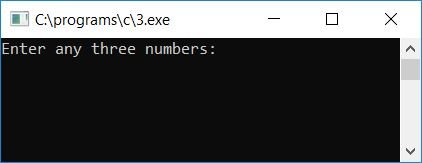Now supply any three numbers as input, say 12, 23, and 11, and then press ENTER to see the following output: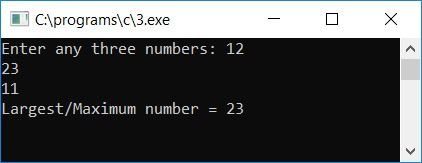#### Program Explained

• Receive any three numbers as input, say num1, num2, and num3.
• Use the ternary operator (?:) to assign the largest value to the variable, say large.
• Here, if the value at num1 is greater than the value at num2,
• and the value at num1 is also greater than the value at num3.
• then num1 will be initialized to large.
• otherwise, num3 will be initialized to large.
• And if num2 is greater than num1,
• and num2 is also greater than num3.
• then num2 will be initialized to large.
• otherwise, num3 will be initialized to large.
• Print the value of variable large, which holds the largest value.

Here we have used a nested ternary operator, which is a ternary operator inside a ternary operator.

## Find the greatest among three numbers using a function

This is another program doing the same job as all the previous programs did. The only difference with previous programs is that this one is created using a user-defined function, findLarge().

```#include<stdio.h>
#include<conio.h>
int findLarge(int, int, int);
int main()
{
int a, b, c, big;
printf("Enter three numbers: ");
scanf("%d%d%d", &a, &b, &c);
big = findLarge(a, b, c);
printf("\nLargest number = %d", big);
getch();
return 0;
}
int findLarge(int num1, int num2, int num3)
{
if(num1>num2)
{
if(num2>num3)
return num1;
else
{
if(num3>num1)
return num3;
else
return num1;
}
}
else
{
if(num2>num3)
return num2;
else
return num3;
}
}```

Here is the final snapshot of the sample run: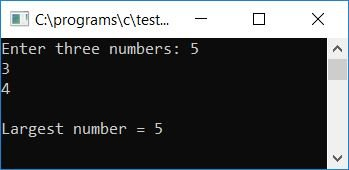Here, the function takes the three given numbers as its argument and will return the largest one, which will be initialized to the variable big inside the main() function. Print the value of "big," which will hold the largest value among the given three numbers. To learn more about function, you can follow a separate tutorial on it.

#### The same program in different languages

C Quiz

« Previous Program Next Program »